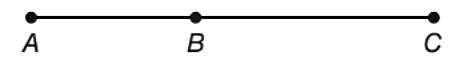Chapter 1.2, Problem 36E### Elementary Geometry for College St...

6th Edition
Daniel C. Alexander + 1 other
ISBN: 9781285195698

#### Solutions

Chapter
Section### Elementary Geometry for College St...

6th Edition
Daniel C. Alexander + 1 other
ISBN: 9781285195698
Textbook Problem
1 views

# In Exercises 35 to 38, with A - B - C on A C ¯ , it follows that A B + B C = A C .Find A B if A C = 25 and B C = 11 .

To determine

To find:

AB for AC=25 and BC=11.

Explanation

Calculation:

Given,

AC=25

BC=11.

AB+BC=AC.

Substitute; AC=25 and BC=11 in the above relation,

AB+11=25

### Still sussing out bartleby?

Check out a sample textbook solution.

See a sample solution

#### The Solution to Your Study Problems

Bartleby provides explanations to thousands of textbook problems written by our experts, many with advanced degrees!

Get Started

#### Find more solutions based on key concepts